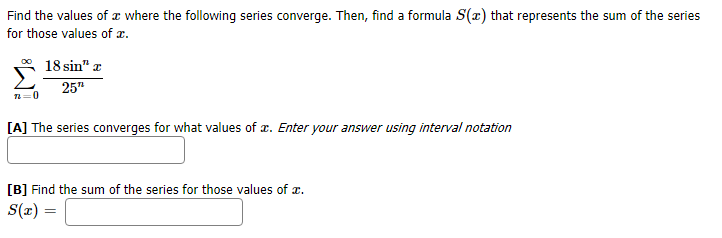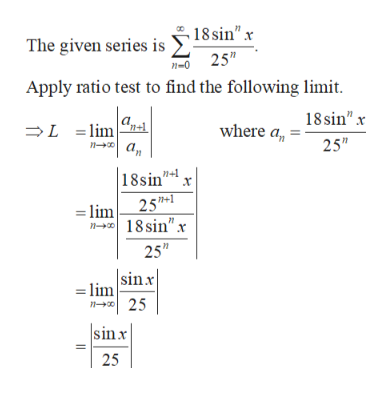# Find the values of where the following series converge. Then, find a formula S(x) that represents the sum of the seriesfor those values ofOO18 sin" r25"0[A] The series converges for what values of r. Enter your answer using interval notation[B] Find the sum of the series for those values of= (x)S

Question
89 viewshelp_outlineImage TranscriptioncloseFind the values of where the following series converge. Then, find a formula S(x) that represents the sum of the series for those values of OO 18 sin" r 25" 0 [A] The series converges for what values of r. Enter your answer using interval notation [B] Find the sum of the series for those values of = (x)S fullscreen
check_circle

Step 1

[A] Obtain the limit of the series as follows.help_outlineImage Transcriptionclose18 sin" x The given series is 25" n-0 Apply ratio test to find the following limit 18sin"x L lim n a where a 25" 18sin" х 25- =lim n-3018 sin"x 25" sinx =lim n 25 sinx 25 fullscreen
Step 2

Obtain the interval where the ser...

### Want to see the full answer?

See Solution

#### Want to see this answer and more?

Solutions are written by subject experts who are available 24/7. Questions are typically answered within 1 hour.*

See Solution
*Response times may vary by subject and question.
Tagged in

### Calculus